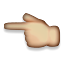## Welcome to the Treehouse Community

Want to collaborate on code errors? Have bugs you need feedback on? Looking for an extra set of eyes on your latest project? Get support with fellow developers, designers, and programmers of all backgrounds and skill levels here with the Treehouse Community!

### Looking to learn something new?

Treehouse offers a seven day free trial for new students. Get access to thousands of hours of content and join thousands of Treehouse students and alumni in the community today.# How to understand counting the binary number system.

How do I better understand the values of the binary numbers while adding them up towards the right?## Perhaps this will help.

Here's one way to decode binary numbers. Make a column for each digit, and starting from the right, put a "1" and then as you move left put double the value of the column next to it. Remember, right-to-left (THIS WAY). You'll get something like this, which is a horizontal version of the helper table shown in the quiz:

```128  64  32  16   8   4   2   1   <-- 8 columns for 8 digits
```

Then, put your binary number on the next line, spread into the columns. Now below that, multiply each top number by the binary digit and put the result below. It's easy, since binary digits are either 1 or 0, so you either put the number on top again or zero.

Then finally, add up all the numbers on the bottom row and that's your answer. Like this:

```128  64  32  16   8   4   2   1   <-- starting columns
0   0   0   0   0   1   1   1   <-- multiply by your binary digits
--- --- --- --- --- --- --- ---
0   0   0   0   0   4   2   1   <-- add these up:  4 + 2 + 1  =  7
```

Also, since zeros in front don't count you can skip them. So in this case we really only needed 3 columns.